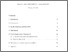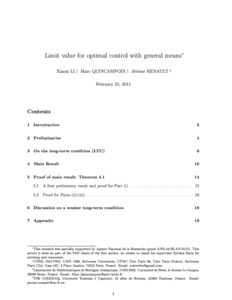Limit Value for Optimal Control with general means

Li, Xiaoxi, Quincampoix, Marc and Renault, Jérôme (2016) Limit Value for Optimal Control with general means. Discrete and Continuous Dynamical Systems, 36 (4). pp. 2113-2132.Preview
Text
Official URL: http://tse-fr.eu/pub/31313

Abstract

We consider optimal control problems where the running cost of the trajectory is evaluated by a probability measure on R. As a particular case, we take the Cesàro average of the running cost over a fixed horizon. The limit of the value with Cesàro average when the horizon tends to infinity is widely studied in the literature. We address the more general question of the existence of a limit for values defined by general evaluations satisfying certain long-term condition. For this aim, we introduce an asymptotic regularity condition for a sequence of probability measures on R. Our main result is that, for any sequence of probability measures on R satisfying this condition, the associated value functions converge uniformly if and only if this family is totally bounded for the uniform norm. As a byproduct, we obtain the existence of a limit value (for general evaluations) for control systems defined on a compact invariant domain and satisfying suitable nonexpansive property.

Item Type: Article English April 2016 Yes Optimal control, limit value, long time average value, general means B- ECONOMIE ET FINANCE TSE-R (Toulouse) UT1 12 Jan 2017 10:24 25 Jul 2019 11:50 oai:tse-fr.eu:31313 http://publications.ut-capitole.fr/id/eprint/22696View Item